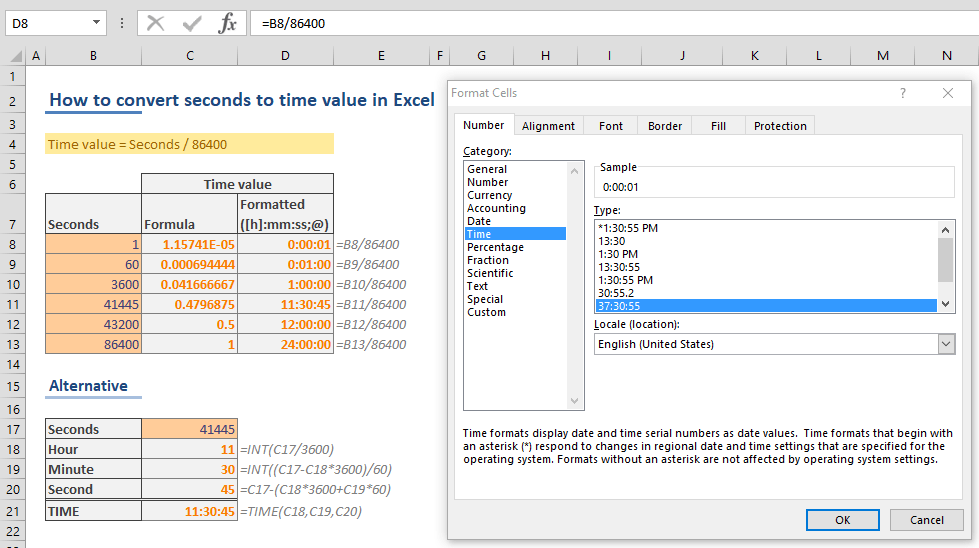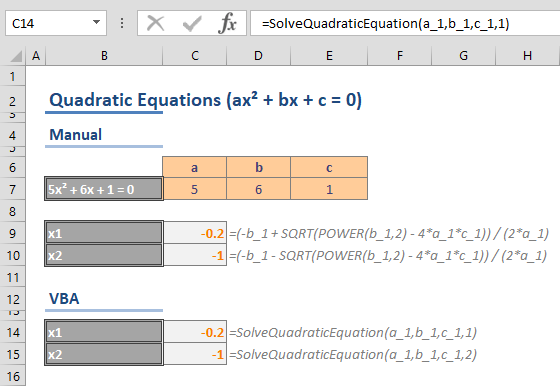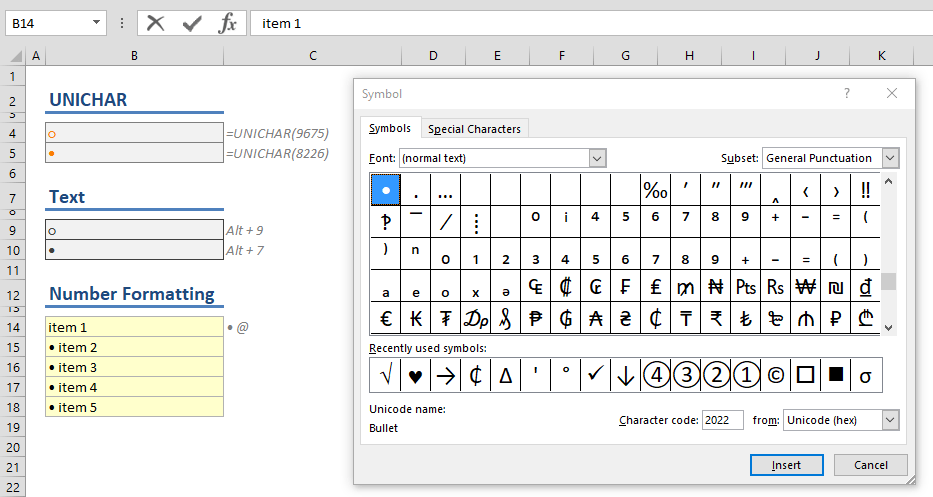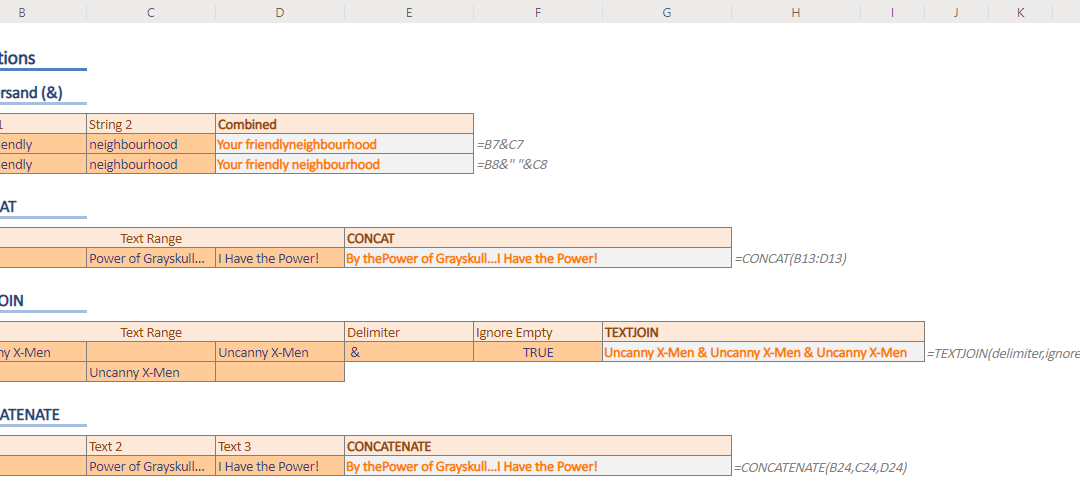## All You Need to Know on How to Create a VBA loop in Excel

A loop is an essential concept for any programming language, and VBA follows the same approach. You can use loops to repeat an action until a specified condition is reached, or move through objects in a group, e.g. all worksheets in a workbook. A loop is an essential...## Control Shift Enter Excel Shortcut (CSE) and Array Formulas

Control Shift Enter Excel shortcut, as known as CSE, is a key combination you can use to apply array functions.CSE abbreviation is also used for array formulas that are entered using the Control + Shift + Enter key combination when entering a formula. Array formulas...## 10 Things You Need to Know About the VLOOKUP Excel Function

In this guide we’re going to go over 10 things you should know about the frequently-used-yet-not-so-easy-to-use VLOOKUP Excel function. Although this guide mainly focuses on the VLOOKUP function, these tips also apply to the HLOOKUP function, which essentially...## How to convert seconds to time value in Excel

When it comes to date and time values, Excel evaluates them a bit differently than how we process them. For Excel, date and time values are numbers in a date or time display. Although this behavior allows making date and time calculations easier, it can be helpful to...## How to compare loans with different durations in Excel

Want to get a loan for a home or vehicle, but can’t decide which option is the best? Use Excel to compare your options and make better decisions! Excel’s PMT function is perfect for this type of analyses. In this article, we’re going to show you how...## How to solve quadratic equations in Excel – Formula and VBA

This is the second part of the how to solve quadratic equations in Excel guide. Here, we are going to be focusing on how to do this manual and VBA method, whereas the first part shows how to do this using the Goal Seek feature. In this guide, we are going to show you...## How to solve quadratic equations in Excel - Goal Seek

A quadratic function is a type of equation that contains a squared variable. It is called quadratic because quad means square in Latin. The quadratic functions usually have a structure like ax² + bx + c = 0, where x represents an unknown variable, and a, b, and c...## How to create bullet points in Excel (•)

Excel is not a word processor software, but you may still want to list things in various parts of your workbooks. Bullet points are great for creating lists, as its the universal indicator of an unordered list. Here, we’re going to cover 4 different ways to...## How to concatenate strings in Excel

Although Excel tasks typically involve working with numbers, you can also manipulate text strings by concatenating them. In this guide, we’re going to show you how to concatenate strings in Excel using the ampersand character and also with the help of other...